# Change Color of Bars in Barchart using ggplot2 in R

• Difficulty Level : Medium
• Last Updated : 06 Jun, 2021

In this article, we are going to see various methods to change the color of a bar chart using ggplot2 in the R programming language.

For creating a simple bar plot we will use the function geom_bar( ).

Syntax:

geom_bar(stat, fill, color, width)

Parameters :

• stat : Set the stat parameter to identify the mode.
• fill : Represents color inside the bars.
• color : Represents color of outlines of the bars.
• width : Represents width of the bars.

Dataset in Use: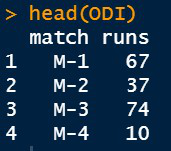Let us first plot a regular barplot to display what a regular plot without any help will be printed.

Example:

## R

 `library``(ggplot2)`` ` `# Inserting data``ODI <- ``data.frame``(match=``c``(``"M-1"``,``"M-2"``,``"M-3"``,``"M-4"``),``                   ``runs=``c``(67,37,74,10))`` ` `# Basic vertical barplot``perf <-``ggplot``(data=ODI, ``aes``(x=match, y=runs))+``  ``geom_bar``(stat=``"identity"``)``perf`` ` `# Horizontal bar plot``perf+``coord_flip``()`

Output: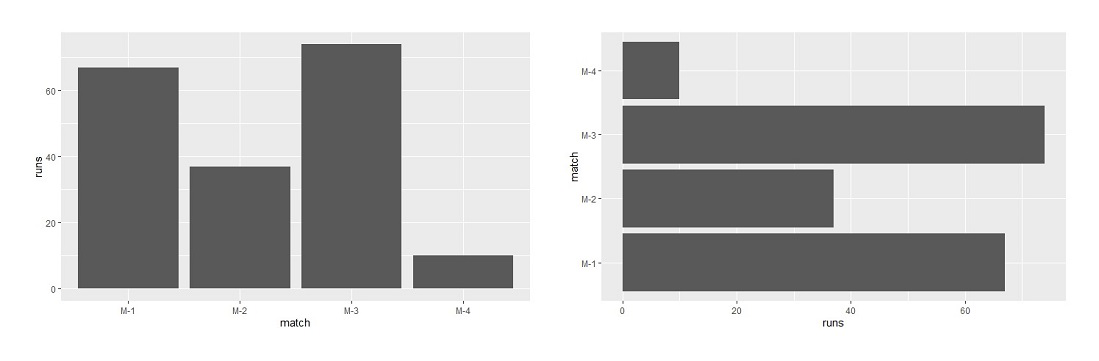Bar Plot

Now let us see how colors are added to the barplot.

### Method 1: Using default colors

• Different fill color

Use the command fill to add color inside the bars. Since, the bars are in different x-axis values we need to assign the x-axis variable to the fill. In our case, match is in the x-axis, so we write fill=match. You need to write this command inside the aes() also known as the quoting function or aesthetic function under the ggplot2 library.

Example:

## R

 `library``(ggplot2)`` ` `# Inserting data``ODI <- ``data.frame``(match=``c``(``"M-1"``,``"M-2"``,``"M-3"``,``"M-4"``),``                   ``runs=``c``(67,37,74,10))`` ` `# Assigning default and different colors to bar plot``perf <-``ggplot``(data=ODI, ``aes``(x=match, y=runs,fill=match))+``  ``geom_bar``(stat=``"identity"``)``perf`

Output: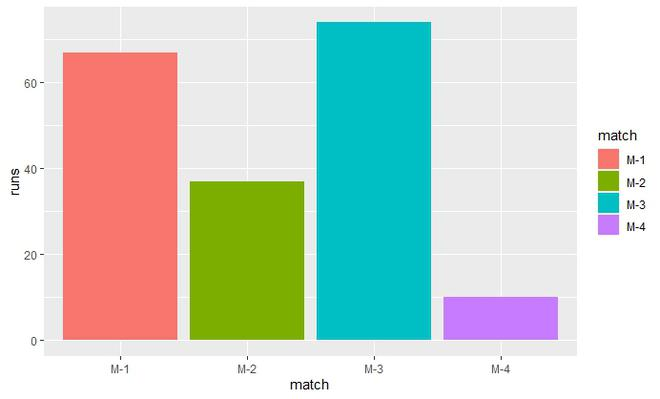• Same fill color

Use the same fill. But this time since we have to assign a single color we will use it inside the geom_bar( ). Then assign the color of your choice to the fill as shown below. The theme is changed because some color plots might not be visible to the default theme in R.

Example:

## R

 `library``(ggplot2)`` ` `# Inserting data``ODI <- ``data.frame``(match=``c``(``"M-1"``,``"M-2"``,``"M-3"``,``"M-4"``),``                   ``runs=``c``(67,37,74,10))`` ` `# Assigning default and same colors to bar plot``perf <-``ggplot``(data=ODI, ``aes``(x=match, y=runs))+``  ``geom_bar``(stat=``"identity"``,fill=``"lightblue"``)+``  ``theme_dark``()``perf`

Output: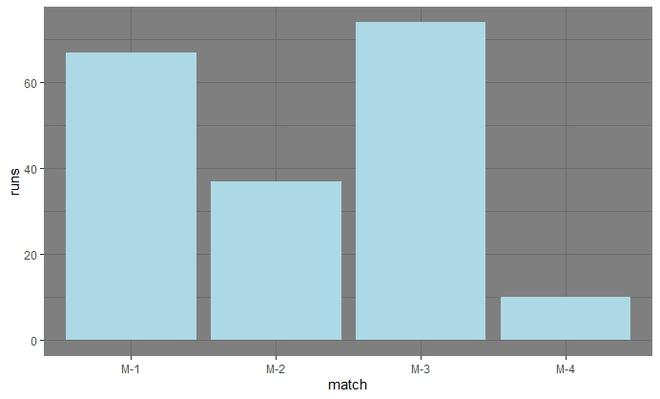• Same outline

Here we need a command color for assigning colors to the outline. Outline colors look good when the fill color is white. It is the property of color because some colors overshadow other colors or if we assign same color to fill and outline both. Since we need the same fill and the same outline we will write these commands inside the geom_bar( ).

Example:

## R

 `library``(ggplot2)`` ` `# Inserting data``ODI <- ``data.frame``(match=``c``(``"M-1"``,``"M-2"``,``"M-3"``,``"M-4"``),``                   ``runs=``c``(67,37,74,10))`` ` `# Assigning default and same outline colors to bar plot``perf <-``ggplot``(data=ODI, ``aes``(x=match, y=runs))+``  ``geom_bar``(stat=``"identity"``,color=``"red"``,fill=``"white"``)+``  ``theme_classic``()``perf`

Output: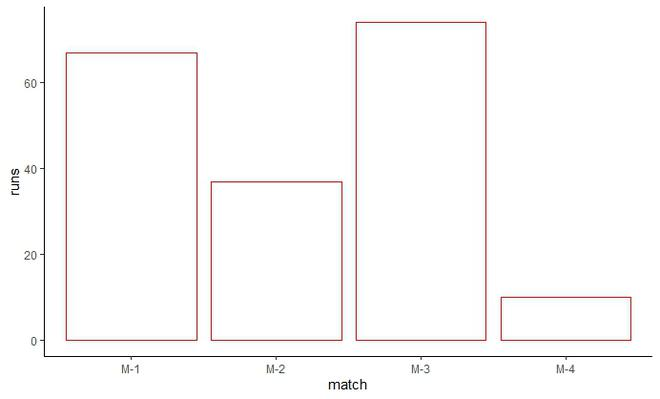• Different Outline

Here we need to provide different outlines. The fill will be the same which is white. The fill will be inside the geom_bar( ). The color will be inside aes( ) under ggplot( ) as it is variable in this case.

Example:

## R

 `library``(ggplot2)`` ` `# Inserting data``ODI <- ``data.frame``(match=``c``(``"M-1"``,``"M-2"``,``"M-3"``,``"M-4"``),``                   ``runs=``c``(67,37,74,10))`` ` `# Assigning default and different outline colors to bar plot``perf <-``ggplot``(data=ODI, ``aes``(x=match, y=runs,color=match))+``  ``geom_bar``(stat=``"identity"``,fill=``"white"``)+``  ``theme_classic``()``perf`

Output: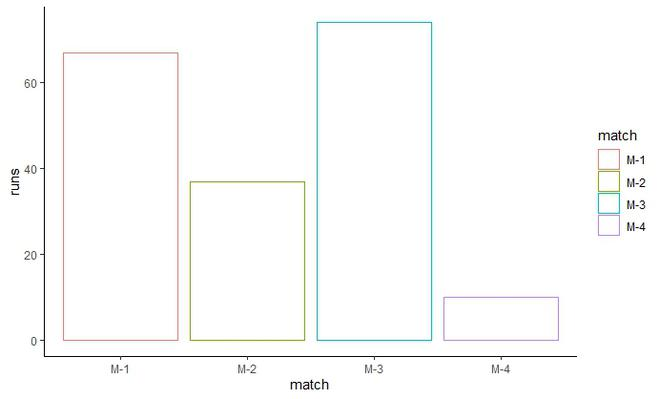### Method 2: Setting color manually

• scale_fill_manual( )

This function is used to provide custom colors. We can either write the color code as “#XXXXXX” or we can directly write the color name as “color_name”. The fill will be inside the ggplot2 as we need to manually assign different colors to the bars. Invoke the function and manually insert a vector which consists of the color code or the color name. The length of the vector should be same as the number of bars.

Example:

## R

 `library``(ggplot2)`` ` `# Inserting data``ODI <- ``data.frame``(match=``c``(``"M-1"``,``"M-2"``,``"M-3"``,``"M-4"``),``                   ``runs=``c``(67,37,74,10))`` ` `# Assigning colors manually ``perf <-``ggplot``(data=ODI, ``aes``(x=match, y=runs,fill=match))+``  ``geom_bar``(stat=``"identity"``)+``  ``scale_fill_manual``(values=``c``(``"#9933FF"``,``                             ``"#33FFFF"``,``                             ``"red"``,``                             ``"darkblue"``))``perf`

Output: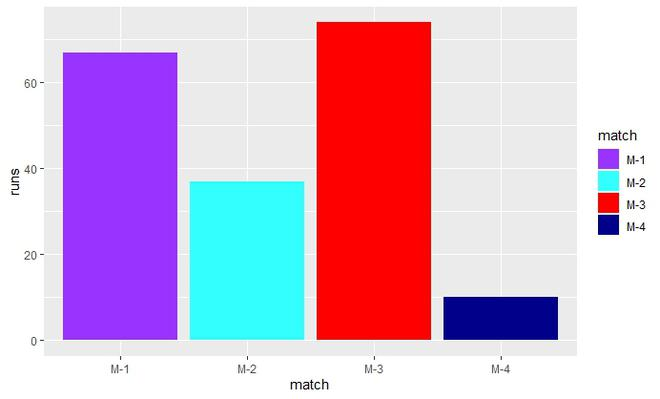Similarly, we can set outlines color manually. Just replace the keyword fill everywhere to color in the above code. The function will now become : scale_color_manual( )

• Gray scale

The function used here is scale_fill_grey( ). The fill is inside the aes( ) as we need different gray scale colors. Invoke the function scale_fill_grey( ) at last.

Example:

## R

 `library``(ggplot2)`` ` `# Inserting data``ODI <- ``data.frame``(match=``c``(``"M-1"``,``"M-2"``,``"M-3"``,``"M-4"``),``                   ``runs=``c``(67,37,74,10))`` ` `# Assigning gray scale colors``perf <-``ggplot``(data=ODI, ``aes``(x=match, y=runs,fill=match))+``  ``geom_bar``(stat=``"identity"``)+``  ``scale_fill_grey``()``perf`

Output: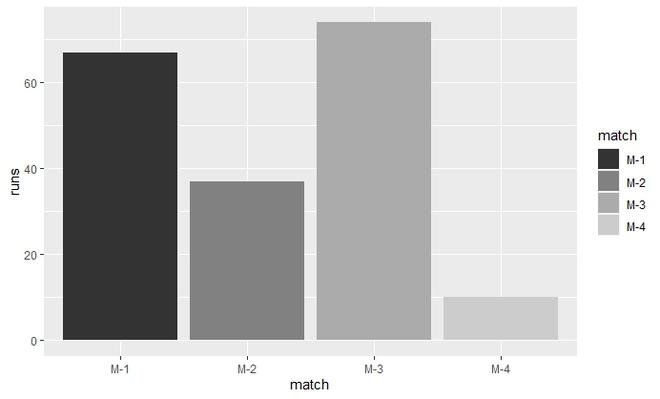My Personal Notes arrow_drop_up